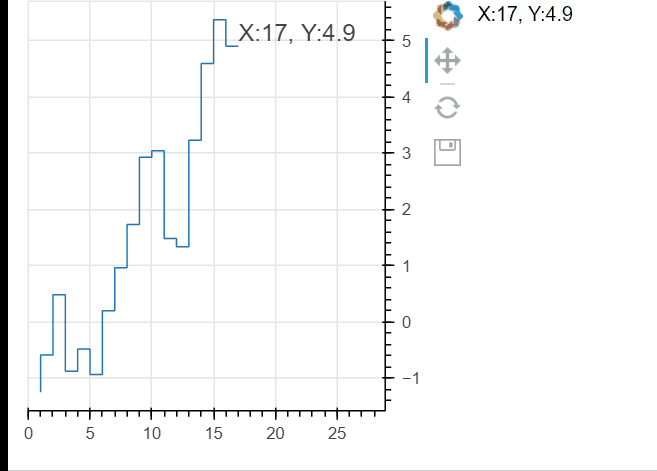# Last value indicator in a plot

Hello!

I am wondering if it is possible recreate a sort of last value indicator in bokeh’s figure. Something similar to the red box on the right axis of this graph which shows the last price

It is essentially a hover tool showing newest value which is always visible and glued to the vertical axis. I am unable to figure out whether that’s actually possible right now?

It’s very possible to get the “last value” and plot it dynamically as a label or something like that. How to get it depends on the glyph you’re using and the datasource that’s driving it. If you can elaborate more on what you’re trying to do with some sandbox code (i.e. minimal reproducible example), I’d be happy to help.

1 Like

Here is basic example

``````from bokeh.layouts import column
from bokeh.plotting import figure, curdoc
from bokeh.models import ColumnDataSource, Plot

import numpy as np

current_x = 0
current_y = 0
def generate_point():
global current_x, current_y
current_x += 1
current_y += np.random.normal()
return dict(x=[current_x], y=[current_y])

def stream_point():
source.stream(generate_point())

p = figure(width=300, height=300, tools="pan,reset,save", y_axis_location="right")
source = ColumnDataSource(data=generate_point())
doc = curdoc()

p.step(source=source)

``````

This should produce a plot with time series moving along in time. The desired outcome would be to have something looking same as in the original question. Some hover tool style box that is glued to the y axis at the height of current_y value and also has current_y written on it.

Just an FYI at least at present there is no option to overlay anything on an axis outside the plot area. You’ll have to settle for a `Label` inside the plot area. 

1. If you wanted to try and get into more detailed things, you could manually position the axis inside the plot area, and then a `Label` could potentially overlay it. But if you are streaming new data you’d have to continuously manually update the axis position on the cross-dimension. I am not really sure that would produce satisfactory results. ↩︎

1 Like

Hopefully this works for you. As mentioned above, you can’t overlay anything on an axis outside the plot area. I’ve presented two options below:

1. use a div outside the plot, PRO: it’s outside the plot CON: it doesn’t move up/down etc
2. use a label inside the plot, PRO: it moves up/down etc, CON: it’s inside the plot

For both, the real trick is to access the last value of the x/y arrays and update the label/glyph with that value.

``````from bokeh.layouts import column,row
from bokeh.plotting import figure, curdoc
from bokeh.models import ColumnDataSource, Plot,Div,Label, Range1d

import numpy as np

current_x = 0
current_y = 0
def generate_point():
global current_x, current_y
current_x += 1
current_y += np.random.normal()
return dict(x=[current_x], y=[current_y])

def stream_point():
source.stream(generate_point())
#update the div text outside the plot
div.text = 'X:'+ str(source.data['x'][-1])+', Y:'+str(np.round(source.data['y'][-1],2))
#update the label x-y coords
lbl.x = source.data['x'][-1]
lbl.y = source.data['y'][-1]
# update the label text
lbl.text = 'X:'+ str(source.data['x'][-1])+', Y:'+str(np.round(source.data['y'][-1],2))
#expand the x_range so the label fits
p.x_range.end= source.data['x'][-1]*1.7

p = figure(width=300, height=300, tools="pan,reset,save", y_axis_location="right")
#make the x_range follow a specific range, not a datasource (because we need to pad it on the right to fit the label)
p.x_range = Range1d(start=0,end=1)
source = ColumnDataSource(data=generate_point())
p.step(source=source)
#make the div and the label, add them to the layout and the figure respectively
div=Div(text='')
lbl=Label(x=current_x,y=current_y,text='X: '+str(current_x)+', Y: '+str(current_y))
doc = curdoc()
``````Another kinda hacky idea (and I could flesh this out for you too if interested) would be to stick this info into a makeshift legend, which would put the value inside the plot located in the upper right or something like that…

Finally my last idea would be some kind of manipulation of the axis tick labels… @Bryan I’m wondering if you can splice in one “special” tick label for the current y value (in addition to the normally calculated tick labels) on the LinearAxis model attached to the figure’s y axis? and if you could even conditionally format that one “special” tick label (like make it bold or red or something. Probably not easily as I’m pretty sure it’s the JS side that decides what tick labels to use, but just another idea…

2 Likes

@gmerritt123 not presently, there were some various issues around more fine-grained formatting of ticks and axes that so far have not gone anywhere:

The main obstacle has been how to allow for specifying a custom format for a given tick, when there is no way to know ahead of time what ticks are where. You can’t just say “make the second tick red” because the second tick can change due to, zoom level, where a user has panned, etc. So, add a hook for some CustomJS “filter” callback that can dynamically figure out what tick to style override? That’s just really complex and user-unfriendly.

At this point I would probably just punt on the idea of individual styles for dynamic ticks, but instead look into ways to let users explicitly specify extra styled ticks or axis annotations independent of and in addition to the ticks that the axis ticker computes.

Thank you so much gmerritt123 ! The label inside the plot is what I have been looking for!

I haven’t thought about doing it this way. It really opens a world of possibilities!

Adding the special tick to the formatter would be really nice, mostly so that we could just pass some flag to bokeh instead of maintaining the xrange ourselves, but this solves my problem as well.

Thank you once more!

1 Like

This topic was automatically closed 90 days after the last reply. New replies are no longer allowed.